## Maths worksheet: estimating on a number line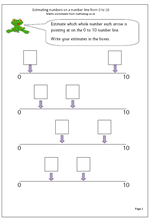By year 2 children should be beginning to understand the vocabulary of estimating, which includes other terms such as roughly, guess how many, about the same as, nearly, close to etc.

This worksheet looks  at estimating a whole number on a number line from 0 to 10. Probably the best technique to use is to try and estimate the middle of the line, which will be 5 and then work either from 0 to 5 or 5 to 10.

Estimating on a number line (pg 1)

## Learning tables: 6x, 7x, 8x, 9x and 10x tables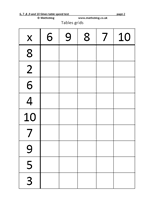This maths worksheet has a tables grid for the 6x, 7x, 8x, 9x and 10x table.
The grid is filled in by taking the numbers across the top with the numbers down the side as shown below.
x 060 7
2 12 14
6 36 42

These are mostly the harder tables to learn, apart from the tens. The nines also have a neat pattern to them; of course remembering that the digits always add up to 9 helps with this table.

There are many different approaches to this type of problem. Some children fill in the answers in strict order, others go through and complete the ones they know off by heart, and then try to work the rest out.
It is a good idea to set a time limit to these to encourage learning them off by heart.

## Maths worksheet: Moving towards standard method for multiplication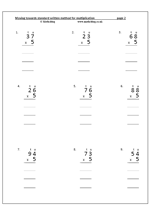This method of multiplication encourages understanding of the process of multiplying a 2-digit number by  a 1-digit number. The questions on this page would not normally be completed using written methods: mental methods should suffice. It would be expected that children could do these ‘in their heads’ from a  knowledge of multiplying by 10 and halving. Also, the Primary Framework for Mathematics does not recommend using these types of written method until Year 3 onwards (7/8 years old).

The method is to multiply the units first, then multiply the tens, finally adding the two together. It is a ‘half-way house’ towards the standard written method, explaining what happens when the tens boundary is crossed during multiplying the units (ie when the units come to more than 10). More details are shown on the worksheet pages below.

The stages are as follows:

36 x 5

Step 1: multiply the units

6 x 5 = 30

Put the 30 in the row below the question, making sure the 3 digit is in the tens column.

Step 2: multiply the tens

3 (tens) x 5  is 15 (tens) = 150.

Put the 150 in the row below, again making sure the units and tens
line up.

Add the units:                      0 + 0 = 0 units
Then add the tens:             3 + 5 = 8 tens
Then add the hundreds:   0 + 1 = 1 hundred

5x moving towards standard multiplication

## Maths worksheet: Rounding to the nearest 10

If the units are 5 or more, round up to the next whole ten.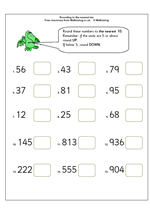Rounding to the nearest ten is a simple process but can cause confusion. The key digit to look at is the units digit. The rule is:

If the units are 5 or more, round up to the next whole ten.

If the units are less than 5, round down to the next whole ten below.

Of course, if the units are zero then there is no need to round. (Some children think they have to go down ten more!)

So, 56 to the nearest whole ten is 60

55 to the nearest whole ten is 60

53 to the nearest 10 is 50

Rounding to the nearest 10

## News: Updating links for the Primary Framework for Maths

You may find a little bit of chaos around the site over the next few days as I am reorganising all the links on the Free Maths Worksheets pages. With the increase in the numbers of worksheets some of the lists are getting quite long, so I am organising them under Year/age group and then subheadings according to the Primary Framework for Maths in England. If you happen to be browsing this section whilst I am updating you may find things suddenly changing or disappearing.

Many apologies for this but it should all be completed soon.

## Year 5 maths worksheet: equivalent fractions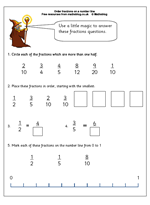Understanding equivalence in fractions is the key to successfully understanding fractions.

This maths worksheet looks at simple equivalence between halves, fifths, tenths etc. The first question asks which fractions are more than a half. The quick way to work this out is to see if the top number (numerator) is more than double the bottom number (denominator) – if it is then it is more than one half.

The second question requires an understanding that a fraction such as three fifths can be changed to an equivalent fraction such as six tenths by multiplying both the numerator and denominator by the same number (in this case 2, but it willl not always be 2).

Equivalent fractions pg 1

## News: fast track teacher training for out of work bankers

The Government are planning for ‘outstanding people’ to qualify as teachers in just six months.
Schools Minister, Jim Knight’ stated today that:
“By cutting the initial teacher training course to six months for the most able candidates, we will make teaching a more attractive choice for experienced people who want to get into the classroom quickly, but need high quality initial teacher training.”
Perhaps the question to ask is what do they mean by ‘experienced people’? A clue comes from another Minister, Liam Byrne, who has said that the six month course is for “good people” with “life experience behind them”.
He goes on to say,
We know there are a lot of fantastic mathematicians, for example, who would have once perhaps gone into the City but now actually might be more interested in a career in teaching.”
Once again, this appears to be devaluing the training that teachers have to do before qualifying, and, unfortunately, having good maths skills suited to work in the Stock Exchange is not necessarily what is needed to teach in tough schools with children who find the subject difficult. Many excellent mathematicians find it impossible to understand other people’s difficulties in the subject.
As Mary Bousted, General Secretary of the Association of Teachers and Lecturers, said,
“It sounds like an employment scheme for unemployed bankers”.

## Year 1 maths worksheet: counting on in tens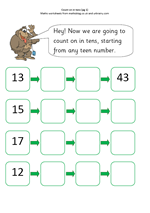Here is a maths worksheet suitable for year 1 children, taken from the great range of maths at urbrainy.com.

When children first learn to count in tens they usually start with whole tens eg 10, 20, 30, 40 etc.

This page takes the concept one step further in that the starting number is not a multiple of 10.

When counting on from 13 children will quickly realise that the units remain the same and that it is the tens digit which is increasing by one  each time.

Count on in tens (pg 1)

## Maths worksheet: adding 4-digit numbers (1)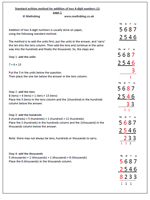Addition of two 4-digit numbers is usually done on paper, using the standard method described on the worksheet. Once addition of HTU has been mastered it is a fairly straightforward step to adding thousands, eg
5 6 8 7 + 2 5 4 6

The key is to keep the numbers in line (tens under tens etc) and to always start with the units. Most children will not have a problem with this and many really enjoy completing sets of sums. It is a good idea to encourage checking by doing the inverse ie subtracting the second number from the answer to give the first number. This checking could be done on a calculator.

## Y3 maths worksheet: time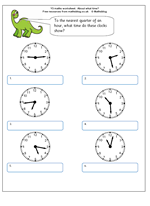This maths worksheet on reading time to the nearest quarter of an hour highlights several issues which create problems for children.

Firstly, on the clock face the hours are clearly numbered but the minutes are not and children need to be able to count on in fives before they can read minutes successfully.

Secondly, when telling someone the time we often approximate, either to the nearest quarter of an hour or the nearest five minutes, even when we can see clearly what the time is to the nearest minute.

Thirdly, when saying the time out or writing it we use several different conventions, as shown on the answer sheet.

Lastly, many children seldom come across this type of clock face, especially if they use digital watches, mobile phones etc., therefore take much longer to work it out – don’t be surprised to find ten year olds unable to read an analogue clock correctly.